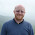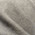## Thursday, 1 August 2013

### Add a bar chart in dc.js

The following post is a portion of the D3 Tips and Tricks book which is free to download. To use this post in context, consider it with the others in the blog or just download the the book as a pdf / epub or mobi .
----------------------------------------------------------
The ubiquitous bar chart is a smart choice if your starting out with crossfilter and dc.js. It's pretty easy to implement and gives a certain degree of instant satisfaction.
The bar chart that we'll create will be a representation of the magnitude of the earthquakes that we have in our dataset. In this respect, what we are expecting to see is the magnitude of the events along the x axis and the number of each such event on the y axis.
It should end up looking a bit like this.

We'll work through adding the chart in stages (and this should work for subsequent charts). Firstly we'll organise a position for our chart on the page using the bootstrap grid set-up. Then we'll name our chart and assign it a chart type. Then we'll create any required dimension and grouping and finally we'll configure the parameters for the chart. Sounds simple right?
1. Position the chart
2. Assign type
3. Dimension and Group
4. Configure chart parameters

### Position the bar chart

We are going to position our bar chart above our data table and we'll actually only make it half the width of our data table so that we can add in another one along side it later.
Just under the line of code that defined the main container for the layout;
```<div class='container' style='font: 12px sans-serif;'>
```
We add in a new row that has two `span6`'s in it (remembering our total is a span of 12 (see the section on bootstrap layout if it's a bit unfamiliar))
```  <div class='row'>
<div class='span6' id='dc-magnitude-chart'>
<h4>Events by Magnitude</h4>
</div>
<div class='span6' id='blank'>
<h4>Blank</h4>
</div>
</div>
```
We've given the first `span6` and ID selector of `dc-magnitude-chart`. So when we we assign our chart that selector, it will automatically appear in that position. We've also put a simple title in place (`<h4>Events by Magnitude</h4>`). The second `span6` is set as blank for the time being (we'll put another bar chart in it later).

### Assign the bar chart type

Here we give our chart it's name (`magnitudeChart`), assign it with a dc.js chart type (in this case `barChart`) and assign it to the ID selector (`dc-magnitude-chart`).
Under the line that assigns the `dataTable` chart type...
```  var dataTable = dc.dataTable("#dc-table-graph");
```
... add in the equivalent for our bar chart.
```  var dataTable = dc.dataTable("#dc-table-graph");
var magnitudeChart = dc.barChart("#dc-magnitude-chart");
```
All done.

### Dimension and group the bar chart data

To set our dimension for magnitude, it's as simple as following the same format as we had previously done for the data table but in this case using the `.mag` variable.
This should go just before the portion of the code that created the data table dimension.
```  var magValue = facts.dimension(function (d) {
return d.mag;
});
```
This dimension (`magValue`) has been set and now has, as its index, each unique magnitude that is seen in the database. This is essentially defining the values on the x axis for our bar chart.
Then we want to group the data by counting the number of events of each magnitude.
```  var magValueGroupCount = magValue.group()
.reduceCount(function(d) { return d.mag; }) // counts
```
This piece of code (which should do directly under the magValue dimension portion), groups (`.group()`) by counting (`.reduceCount`) all of the magnitude values (`function(d) { return d.mag; })`) and assigns it to the `magValueGroupCount` variable. This has essentially defined the values for the y axis of our bar chart (the number of times each magnitude occurs).

### Configure the bar chart parameters

There are lots of parameters that can be configured, and if the truth be told, I haven't explored all of them or, in some cases, worked out exactly how they work.
However, the best way to learn is by doing, so here is the block of code for configuring the bar chart. This should go just before the block that configures the dataTable.
```  magnitudeChart.width(480)
.height(150)
.margins({top: 10, right: 10, bottom: 20, left: 40})
.dimension(magValue)
.group(magValueGroupCount)
.transitionDuration(500)
.centerBar(true)
.gap(65)
.filter([3, 5])
.x(d3.scale.linear().domain([0.5, 7.5]))
.elasticY(true)
.xAxis().tickFormat();
```
That should be it. With the addition of this portion of the code, you should have a functioning visualization that can be filtered dynamically. Just check to make sure that everything is working properly and we'll go through some of the configuration options to see what they do.
Your web page should look a little like this;
The configuration options start by declaring the name of the chart (`magnitudeChart`) and setting the height and width of the chart.
```  magnitudeChart.width(480)
.height(150)
```
In the case of our example I have selected the width based on the default size for a `span6` grid segment in bootstrap and adjusted the height to make it look suitable.
Then we have our margins set up.
```    .margins({top: 10, right: 10, bottom: 20, left: 40})
```
Nothing too surprising there although the left margin is slightly larger to allow for larger values on the y axis to be represented without them getting clipped.
Then we define which dimension and grouping we will use.
```    .dimension(magValue)
.group(magValueGroupCount)```
I like to think of this section as the .dimension declaration being the x axis and the .group declaration being the y axis. This just helps me get the graph straight in my head before it's plotted.
The `.transitionDuration` setting defines the length of time that any change takes to be applied to the chart as it adjusts.
```    .transitionDuration(500)
```
Then we ensure that the bar for the bar graph is centred on the ticks on the x axis.
```    .centerBar(true)
```
Without this (`true` is not the default), the graph will look like slightly odd.
The setting of the gap between the bars is accomplished with the following setting;
`    .gap(65) `

I will admit that I still don't quite understand how this setting works exactly, but I can get it to do what I want with a little trial and error.
For instance, I would expect that `.gap(2)` would have the effect of producing a gap of 2 pixels between the bars. But this would be the result for our graph if I have that set.
If you select a portion of the graph you will see some strange things going on. That appears to be as a result of the bars being too wide for the graph.
Setting the gap for a bar graph is a pretty tricky thing to do (progromatically), and I can see why it would throw some strange results. The way around this and the way to find the ideal `.gap` setting is to set the `.gap`value high and then reduce it till it's right.
For instance, if we set it to 100 (`.gap(100)`) we will get the following result.
Then we just keep backing the values off till we reach an acceptable chart on the screen.
In the case of our example, it's `.gap(65)`.
I have added in the next setting more because I want you to know it exists, rather than wanting to use it in this example.
```    .filter([3, 5])
```

Setting the `.filter` configuration will load the graph with a portion of it pre-selected. If you omit this parameter, the entire graph is selected by default. In most cases that I can think of, that is what I would start with.
We can set the range of values presented in our graph by defining the domain (in the same way as for d3.js).
```    .x(d3.scale.linear().domain([0.5, 7.5]))
```
The next parameter sets the y axis to adjust dynamically as the filtered data is returned.
```    .elasticY(true)
```
The final parameter that we set is to format the values on the x axis.

```    .xAxis().tickFormat();
```
And that's it! A bar graph added to your visualization with full dynamic control.

### Just one more thing...

Just another snippet that could be useful. In the section where we set up our group to count the number of instances of individual magnitudes we had;
```  var magValueGroupCount = magValue.group()
.reduceCount(function(d) { return d.mag; }) // counts
```
We could have just as easily summed the magnitude values instead of counting them by using `.reduceSum `instead of `.reduceCount`. This has the effect of increasing the value on the y axis (as the sum of the magnitudes would have been greater than the count) like so
The reason I mention it is that summing the numeric value would be useful in many circumstances (file size or packet size or similar).

The description above (and heaps of other stuff) is in the D3 Tips and Tricks book that can be downloaded for free (or donate if you really want to :-)).

1.Congrats on being the second hit in google for "dc.js example"!
Kudos for braving this trail.

1.Lol! Is that right? I'm sure that there will be many more good examples to come from dc.js. It's the only way I could find to implement crossfilter in a way that I could understand. I therefore imagine that there will be a few more souls in the same boat as I. Nick Zhu has done some great work here to make it accessible to a wider audience.

2.I found a slight problem with this, from the example in the book anyway. When having an initial filter the table underneath isn't populated until the selection is altered. Wondered if you found that happened?

1.Interesting! I can't say that I've come across this before. It might be because of the versions of dc.js / crossfilter and d3.js? Possibly some subtle interaction causing an unwanted 'feature'?

3.Great blog/book by the way!

1.2.Hi. It's looking great. I wanted to redraw the graph if I click the bars of the chart and also the same should be replicated in the dependent charts. I tried on the same with renderlet but not able to achieve it. Do you have any idea/brief note on this? You can update it in your blog.

3.Hi, Sorry for the delay in replying. I don't have any familiarity with renderlet, so I can't really advise either way.

4.Hey! How can we add xAxisLabels within dc.js? I tried using .xAxisLabels('Magnitude ') but it din't work..
Here's the complete code:

magnitudeChart.width(480)
.height(200)
.margins({top: 10, right: 10, bottom: 20, left:40})
.dimension(magValue) // x-axis
.group(magValuegroup) //y-axis
.transitionDuration(500)
.centerBar(true)
.gap(65)
.filter([3, 5])
.x(d3.scale.linear().domain([0.5, 7.5]))
.elasticY(true)
.xAxisLabel('Magnitude')
.yAxisLabel('jhg')
.xAxis().tickFormat();

1.Sorry for the late reply. I'm not entirely sure what the answer will be, but it's possible that it could be order dependant. Try swapping the order of the x axis label call to above the xAxis call.

5.Hi, Thanks for the great article. I followed the exact same steps and i'm encountering an issue. It is throwing an error saying "Uncaught TypeError: group.all is not a function".

Can someone please guide me on what could be the issue here?

Thanks,
V

6.Hi, I want values of x-axis in the range of 2 for example 2, 4, 6... Actually by default my x-axis values range getting in 1,2,3,4, ....14.
Could you please tell us how can I achieve this? I will be grateful for any help you can provide.

1.Hmm... That might be slightly difficult from memory as I believe that the axes function is abstracted by dc.js. I would be looking at how necessary it was for the specific values. I'm sorry that's not much help

7.Nice post... here are some more crossfilter links... http://robertjliguori.blogspot.com/2016/12/interactive-data-visualization-with.html